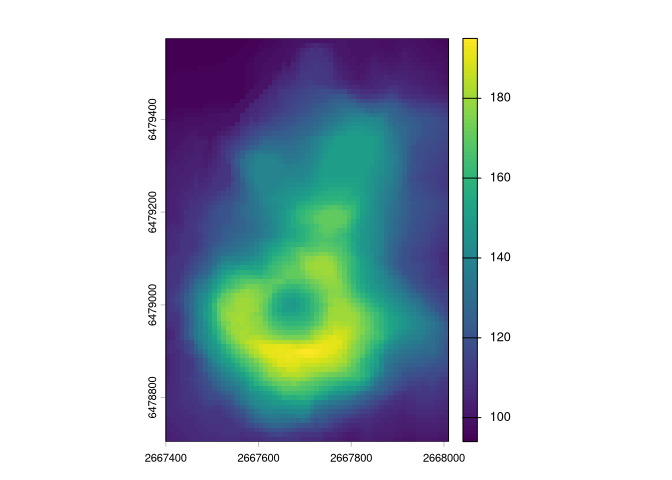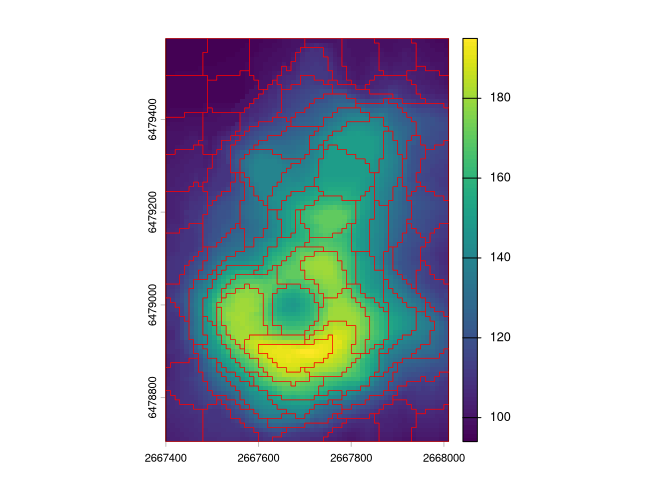The goal of supercells is to utilize the concept of superpixels to a variety of spatial data. This package works on spatial data with one variable (e.g., continuous raster), many variables (e.g., RGB rasters), and spatial patterns (e.g., areas in categorical rasters). It is based on the SLIC algorithm (Achanta et al. (2012)), and readapts it to work with arbitrary dissimilarity measures.

## Installation

You can install the released version of supercells from CRAN with:

install.packages("supercells")

You can install the development version from GitHub with:

install.packages("supercells", repos = "https://nowosad.r-universe.dev")

## Example

library(supercells)
library(terra)
#> terra 1.5.20
library(sf)
#> Linking to GEOS 3.9.2, GDAL 3.3.2, PROJ 8.2.1; sf_use_s2() is TRUE
vol = rast(system.file("raster/volcano.tif", package = "supercells"))
plot(vol)vol_slic1 = supercells(vol, k = 50, compactness = 1)
plot(vol)
plot(st_geometry(vol_slic1), add = TRUE, lwd = 0.2)## Documentation

See the package’s vignettes: